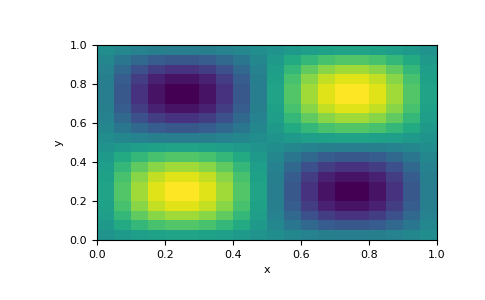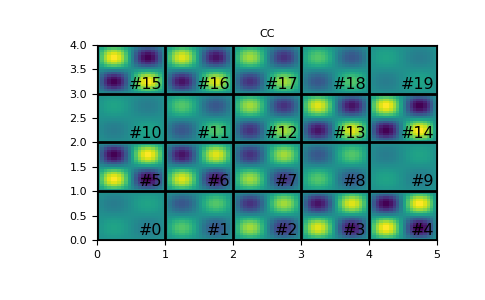# discretize.mixins.InterfaceMPL.plot_image¶

InterfaceMPL.plot_image(v, v_type='CC', grid=False, view='real', ax=None, clim=None, show_it=False, pcolor_opts=None, stream_opts=None, grid_opts=None, range_x=None, range_y=None, sample_grid=None, stream_thickness=None, stream_threshold=None, **kwargs)[source]

Plots quantities defined on a given mesh.

This method is primarily used to plot models, scalar quantities and vector quantities defined on 2D meshes. For 3D `discretize.TensorMesh` however, this method will plot the quantity for every slice of the 3D mesh.

Parameters
v`numpy.ndarray`

Gridded values being plotted. The length of the array depends on the quantity being plotted; e.g. if the quantity is a scalar value defined on mesh nodes, the length must be equal to the number of mesh nodes.

v_type{‘CC’,’CCV’, ‘N’, ‘F’, ‘Fx’, ‘Fy’, ‘Fz’, ‘E’, ‘Ex’, ‘Ey’, ‘Ez’}

Defines the input parameter v.

view{‘real’, ‘imag’, ‘abs’, ‘vec’}

For complex scalar quantities, options are included to image the real, imaginary or absolute value. For vector quantities, view must be set to ‘vec’.

ax`matplotlib.axes.Axes`, `optional`

The axes to draw on. None produces a new Axes.

clim`tuple` `of` `float`, `optional`

length 2 tuple of (vmin, vmax) for the color limits

range_x, range_y`tuple` `of` `float`, `optional`

length 2 tuple of (min, max) for the bounds of the plot axes.

pcolor_opts`dict`, `optional`

Arguments passed on to `pcolormesh`

gridbool, `optional`

Whether to plot the edges of the mesh cells.

grid_opts`dict`, `optional`

If `grid` is true, arguments passed on to `plot` for grid

sample_grid`tuple` `of` `numpy.ndarray`, `optional`

If `view` == ‘vec’, mesh cell widths (hx, hy) to interpolate onto for vector plotting

stream_opts`dict`, `optional`

If `view` == ‘vec’, arguments passed on to `streamplot`

stream_thickness`float`, `optional`

If `view` == ‘vec’, linewidth for `streamplot`

stream_threshold`float`, `optional`

If `view` == ‘vec’, only plots vectors with magnitude above this threshold

show_itbool, `optional`

Whether to call plt.show()

numberingbool, `optional`

For 3D `TensorMesh` only, show the numbering of the slices

annotation_color`Color` or `str`, `optional`

For 3D `TensorMesh` only, color of the annotation

Examples

2D `TensorMesh` plotting

```>>> from matplotlib import pyplot as plt
>>> import discretize
>>> import numpy as np
>>> M = discretize.TensorMesh([20, 20])
>>> v = np.sin(M.gridCC[:, 0]*2*np.pi)*np.sin(M.gridCC[:, 1]*2*np.pi)
>>> M.plot_image(v)
>>> plt.show()
```3D `TensorMesh` plotting

```>>> import discretize
>>> import numpy as np
>>> M = discretize.TensorMesh([20, 20, 20])
>>> v = np.sin(M.gridCC[:, 0]*2*np.pi)*np.sin(M.gridCC[:, 1]*2*np.pi)*np.sin(M.gridCC[:, 2]*2*np.pi)
>>> M.plot_image(v, annotation_color='k')
>>> plt.show()
```

(png, pdf)